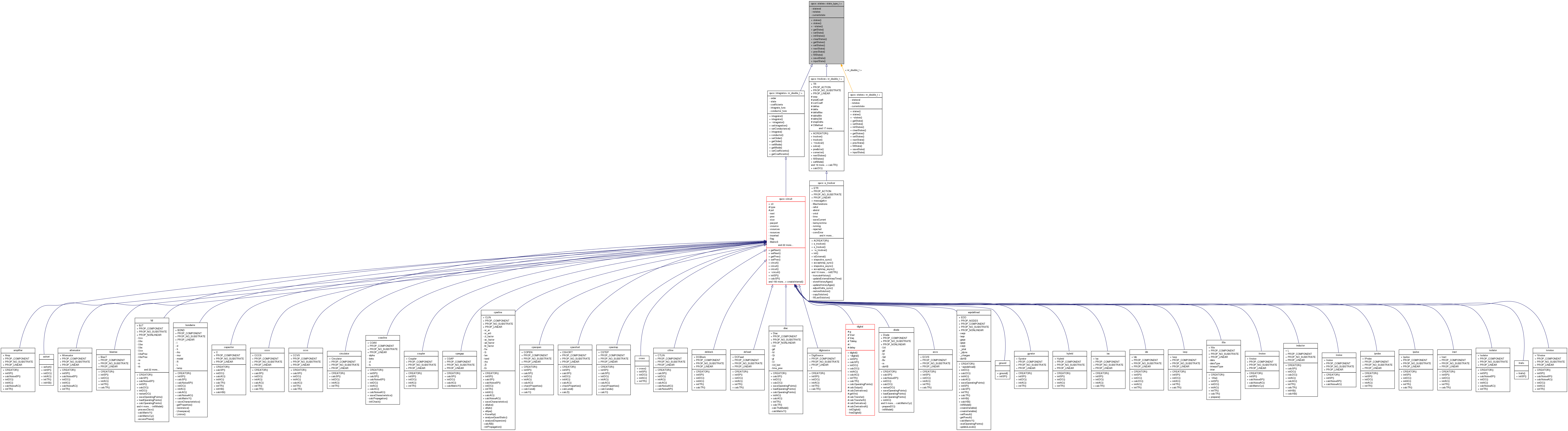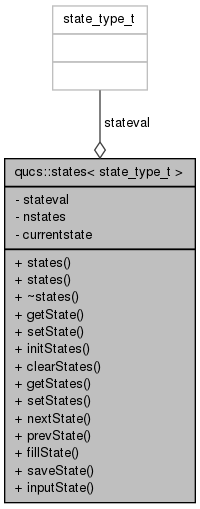Qucs-core  0.0.19
qucs::states< state_type_t > Class Template Reference

template class for storing state variables. More...

`#include <states.h>`

Inheritance diagram for qucs::states< state_type_t >:[legend]
Collaboration diagram for qucs::states< state_type_t >:[legend]

Public Member Functions

states ()
states (const states &)
~states ()
state_type_t getState (int, int n=0)
void setState (int, state_type_t, int n=0)
void initStates (void)
void clearStates (void)
int getStates (void)
void setStates (int n)
void nextState (void)
void prevState (void)
void fillState (int, state_type_t)
void saveState (int, state_type_t *)
void inputState (int, state_type_t *)

Private Attributes

state_type_t * stateval
int nstates
int currentstate

Detailed Description

template<class state_type_t> class qucs::states< state_type_t >

template class for storing state variables.

This class is used for storing sets of states for use by the transient integrators.

Definition at line 38 of file states.h.

Constructor & Destructor Documentation

template<class state_type_t >
 qucs::states< state_type_t >::states ( )

Definition at line 40 of file states.cpp.

template<class state_type_t >
 qucs::states< state_type_t >::states ( const states< state_type_t > & c )

Definition at line 50 of file states.cpp.

template<class state_type_t >
 qucs::states< state_type_t >::~states ( )

Definition at line 67 of file states.cpp.

Member Function Documentation

template<class state_type_t >
 void qucs::states< state_type_t >::clearStates ( void )

Definition at line 88 of file states.cpp.

template<class state_type_t>
 void qucs::states< state_type_t >::fillState ( int state, state_type_t val )

Definition at line 132 of file states.cpp.

template<class state_type_t >
 state_type_t qucs::states< state_type_t >::getState ( int state, int n = `0` )

Definition at line 99 of file states.cpp.

template<class state_type_t>
 int qucs::states< state_type_t >::getStates ( void ) ` [inline]`

Definition at line 51 of file states.h.

template<class state_type_t >
 void qucs::states< state_type_t >::initStates ( void )

Definition at line 75 of file states.cpp.

template<class state_type_t>
 void qucs::states< state_type_t >::inputState ( int state, state_type_t * values )

Definition at line 159 of file states.cpp.

template<class state_type_t >
 void qucs::states< state_type_t >::nextState ( void )

Definition at line 117 of file states.cpp.

template<class state_type_t >
 void qucs::states< state_type_t >::prevState ( void )

Definition at line 124 of file states.cpp.

template<class state_type_t>
 void qucs::states< state_type_t >::saveState ( int state, state_type_t * values )

Definition at line 146 of file states.cpp.

template<class state_type_t>
 void qucs::states< state_type_t >::setState ( int state, state_type_t val, int n = `0` )

Definition at line 109 of file states.cpp.

template<class state_type_t>
 void qucs::states< state_type_t >::setStates ( int n ) ` [inline]`

Definition at line 52 of file states.h.

Field Documentation

template<class state_type_t>
 int qucs::states< state_type_t >::currentstate` [private]`

Definition at line 65 of file states.h.

template<class state_type_t>
 int qucs::states< state_type_t >::nstates` [private]`

Definition at line 64 of file states.h.

template<class state_type_t>
 state_type_t* qucs::states< state_type_t >::stateval` [private]`

Definition at line 63 of file states.h.

The documentation for this class was generated from the following files: